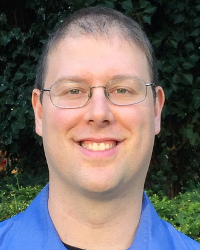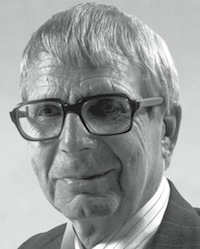Ahlfors Lecture series

Speaker and Program

 Jacob Fox (Stanford University)
 September 17, 2015: Lecture I 4:15-5:15 PM in SC Hall A The Graph Regularity Method It is a fundamental problem to understand the structure of large graphs, as it can yield critical insights into topics ranging from the spread of diseases to how the brain works. Szemerédi's regularity lemma gives a rough structural result for all graphs. It is one of the most powerful tools in graph theory, with many applications in combinatorics, number theory, discrete geometry, and computer science. Roughly speaking, it says that every graph can be partitioned into a small number of parts such that between almost all pairs of parts the graph is random-like. Variants of the regularity lemma have since been established with many further applications. In this talk, I will introduce the regularity method and its applications, and survey recent progress in understanding the quantitative aspects of these results. September 18, 2015: Lecture II 4:15-5:15 PM in SC Hall D Sparse Regularity and Prime Patterns The celebrated Green-Tao theorem states that there are arbitrarily long arithmetic progressions in the primes. In this talk, I will explain the ideas of the proof and recent joint work with David Conlon and Yufei Zhao simplifying the proof. A key ingredient in the proof of the Green-Tao theorem is a relative Szemerédi theorem, which says that any subset of a pseudorandom set of integers of positive relative density contains long arithmetic progressions. Our main advance is a simple proof of a strengthening of the relative Szemerédi theorem, showing that a much weaker pseudorandomness condition is sufficient. The key component in our proof is an extension of the regularity method to sparse hypergraphs.This is a lecture series in honor of Lars Ahlfors (1907-1996) who was William Caspar Graustein Professor of Mathematics at Harvard University from 1946 to 1977. Ahlfors won the fields medal in 1936 and the Wolf prize in 1981.

History:

Ahlfors Lecture Series, 2009 Ahlfors Lecture Series, 2010 Ahlfors Lecture Series, 2011 Ahlfors Lecture Series, 2012 Ahlfors Lecture Series, 2013 Ahlfors Lecture Series, 2014